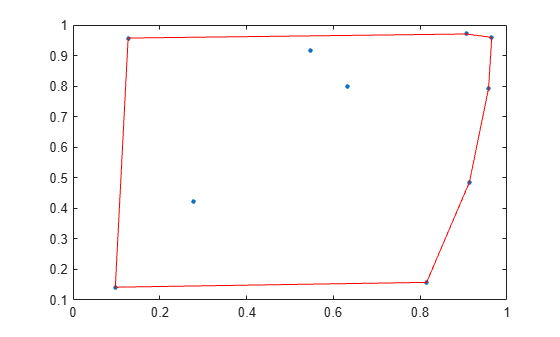convexHull

Convex hull of Delaunay triangulation

Description

example

C = convexHull(DT) returns the vertices of the convex hull of a Delaunay triangulation.

example

[C,v] = convexHull(DT) also returns the area or volume bounded by the convex hull.

Examples

collapse all

Compute and plot the convex hull of a 2-D Delaunay triangulation.

Create a Delaunay triangulation from a set of 2-D points.

rng default;
x = rand([10,1]);
y = rand([10,1]);
DT = delaunayTriangulation(x,y);

Compute the convex hull.

C = convexHull(DT);

Plot the triangulation and highlight the convex hull in red.

plot(DT.Points(:,1),DT.Points(:,2),'.','MarkerSize',10)
hold on
plot(DT.Points(C,1),DT.Points(C,2),'r')Compute and plot the convex hull of a 3-D Delaunay Triangulation.

Create a Delaunay triangulation from a 3-D set of points.

rng('default');
P = rand([25,3]);
DT = delaunayTriangulation(P);

Compute the convex hull and the volume bounded by the convex hull.

[C,v] = convexHull(DT);

Display the volume and plot the convex hull.

v
v = 0.3943
trisurf(C,DT.Points(:,1),DT.Points(:,2),DT.Points(:,3), ...
'FaceColor','cyan')Input Arguments

collapse all

Delaunay triangulation, specified as a scalar delaunayTriangulation object.

Data Types: delaunayTriangulation

Output Arguments

collapse all

Convex hull vertices, returned as a column vector or matrix of vertex IDs.

• When DT is a 2-D triangulation, C is a column vector containing the sequence of vertex IDs around the convex hull. The vertex IDs are the row numbers of the vertices in the Points property.

• When DT is 3-D triangulation, C is a 3-column matrix containing the connectivity list of triangle vertices in the convex hull.

Data Types: double

Area or volume of the convex hull, returned as a scalar.

Data Types: double